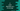# C program to print alternate numbers of an user input array### C program to print alternate numbers of a user input array :

In this tutorial, we will learn how to print alternate numbers of an array . User will enter the total count of numbers the array will hold. Then the program will take input for each position of that array. Let’s take a look into the program first :

### C program :

``````#include <stdio.h>

int main()
{
//1
int i;
int size;

//2
printf("How many numbers you want to enter : ");
scanf("%d", &size);

//3
int array[size];

//4
for (i = 0; i < size; i++)
{
printf("Enter number %d : ", i + 1);
scanf("%d", &array[i]);
}

//5
printf("Alternate elements of the input array : \n");
for (i = 0; i < size; i += 2)
{
printf("%d ", array[i]);
}
}``````

It will print output as like below:

``````How many numbers you want to enter : 10
Enter number 1 : 1
Enter number 2 : 2
Enter number 3 : 3
Enter number 4 : 4
Enter number 5 : 5
Enter number 6 : 6
Enter number 7 : 7
Enter number 8 : 8
Enter number 9 : 9
Enter number 10 : 10
Alternate elements of the input array :
1 3 5 7 9``````

### Explanation :

The commented numbers in the above program denotes the step number below :

1. Create one variable i to use in the loop. Also, create another variable size to store the size of the array.
2. Ask the user how many numbers he is entering. Store this value in variable size.
3. Create one array with a size equal to the user-given value.
4. Run one for loop to read the contents for the array. Read each element one by one and store these in the array.
5. Use one more for loop and print the alternate elements of the array. In this loop, we are incrementing the value of i by 2 like i += 2 . Not i++. So, this loop will print the alternate numbers in the array.

### Sample Output :

``````How many numbers you want to enter : 10
Enter number 1 : 1
Enter number 2 : 2
Enter number 3 : 3
Enter number 4 : 4
Enter number 5 : 5
Enter number 6 : 6
Enter number 7 : 7
Enter number 8 : 8
Enter number 9 : 9
Enter number 10 : 10
Alternate elements of the input array :
1
3
5
7
9
How many numbers you want to enter : 10
Enter number 1 : 10
Enter number 2 : 9
Enter number 3 : 8
Enter number 4 : 7
Enter number 5 : 6
Enter number 6 : 5
Enter number 7 : 4
Enter number 8 : 3
Enter number 9 : 2
Enter number 10 : 1
Alternate elements of the input array :
10
8
6
4
2``````